# Carathéodory interpolation

Jump to: navigation, search

Let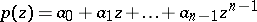be a polynomial of degree at most. Let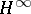be the Hardy space (cf. Hardy spaces) formed by the set of all analytic functionsin the open unit disc whose-norm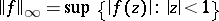is finite. One says thatis an interpolant of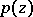ifis a function inandare the firstTaylor coefficients offor, that is,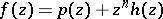for somein(cf. also Taylor series).

The Carathéodory interpolation problem is to find the set of all interpolantsofsatisfying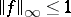. Of course, this set can be empty. Let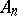be the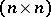lower triangular Toeplitz matrix defined by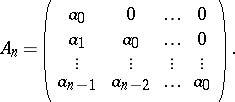Then there exists a solution of the Carathéodory interpolation problem if and only if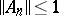. Moreover, there exists a unique solution of the Carathéodory interpolation problem if and only if. In this case the unique interpolantofsatisfyingis a Blaschke product.

The Schur method for solving the Carathéodory interpolation problem [a1], [a2] is based on the Möbius transformation (cf. Fractional-linear mapping)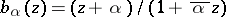where. By recursively unravelling this Möbius transformation, I. Schur discovered thatuniquely determines and is uniquely determined by, whereforms a sequence of complex numbers now referred to as the Schur numbers, or reflection coefficients, for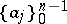. The Schur algorithm is a computational procedure, discovered by Schur, which computes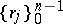from, or vice versa, in about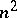computations. Moreover,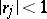for allif and only if. In this case the set of all solutionsof the Carathéodory interpolation problem is given bywhere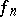is an arbitrary function insatisfying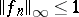. Furthermore,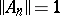if and only iffor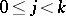and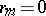for. In this case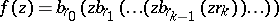is the unique solution of the Carathéodory interpolation problem. If the reflection coefficientsdo not satisfy any one of the previous conditions, then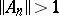and there is no solution of the Carathéodory interpolation problem; see [a4] for further details.

The Schur numbers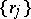are precisely the reflection coefficients which naturally occur in certain inverse scattering problems for layered media in geophysics. Therefore, the Schur algorithm plays an important role in geophysics and marine seismology, see [a3], [a4], [a5]. Finally, it has been noted that the Schur algorithm can also be used to obtain a Routh or Jury test for the open unit disc, that is, the Schur algorithm can be used to determine whether or not a polynomialhas all its roots inside the open unit disc without computing the zeros of; see [a2], [a4].